﻿ Experimental Analysis of Friction Effects Affecting the Snake Robot Segment during Rectilinear Locomotion

### Experimental Analysis of Friction Effects Affecting the Snake Robot Segment during Rectilinear Locom...

Ivan Virgala, Alexander Gmiterko, Peter FrankovskýOPEN ACCESSPEER-REVIEWED

## Experimental Analysis of Friction Effects Affecting the Snake Robot Segment during Rectilinear Locomotion

Ivan Virgala1,, Alexander Gmiterko1, Peter Frankovský1

1Department of Applied Mechanics and Mechatronics, Technical University of Košice / Faculty of Mechanical Engineering, Košice, Slovakia

### Abstract

The paper deals with analysis of rectilinear motion of the snake robot. Considering that snake robot locomotion is based on friction forces between robot and its environment, the basic friction models are introduced. For study the experimental snake robot LocoSnake is used. At first, by mechanic laws the propulsive forces affecting segment of snake robot are determined. By means of mathematical analysis the average velocity of snake robot is derived. Since the number of snake robot segments has influence on robot average velocity, the optimal number of segments for maximum velocity is derived. In the next section the influence of dry and viscous friction on moving segment are described. At last the experimental analysis of three kinds of surfaces are done, namely dry friction, viscous friction using oil and viscous friction using lubricant. The measurements by means of measuring I/O card MF624 was done. In the conclusion the differences between these surfaces are described and discussed. The contribution of the paper is determination of influence of surface change on the snake robot rectilinear locomotion.

### At a glance: Figures

12345
Prev Next

• Virgala, Ivan, Alexander Gmiterko, and Peter Frankovský. "Experimental Analysis of Friction Effects Affecting the Snake Robot Segment during Rectilinear Locomotion." American Journal of Mechanical Engineering 1.7 (2013): 412-416.
• Virgala, I. , Gmiterko, A. , & Frankovský, P. (2013). Experimental Analysis of Friction Effects Affecting the Snake Robot Segment during Rectilinear Locomotion. American Journal of Mechanical Engineering, 1(7), 412-416.
• Virgala, Ivan, Alexander Gmiterko, and Peter Frankovský. "Experimental Analysis of Friction Effects Affecting the Snake Robot Segment during Rectilinear Locomotion." American Journal of Mechanical Engineering 1, no. 7 (2013): 412-416.

 Import into BibTeX Import into EndNote Import into RefMan Import into RefWorks

### 1. Introduction

There are a several kinds of mechanisms which arose by inspiration from nature. One of them is snake robot which has very flexible and adaptive kinematic structure which allows it to moves through very rough and inaccessible environments. The snake robots are mechanisms which from many same segments are composed. They are able to move on the land and in the water as well.

Snake robots are inspired by biological snakes. Biological snake moves by four basic locomotion modes, namely lateral undulation, concertina, sidewinding and rectilinear locomotion [1, 2]. Snake robots are suitable for many tasks like search and rescue operations, inspections of hard-to-reach areas, medicine operations, applications in military etc [2, 3, 4]. Our work group to the pipe inspection tasks is focused.

For pipe inspection tasks are suitable only concertina and rectilinear locomotion because they do not require large place for its locomotion. The advantage of snake robot is its flexibility to the changes in environment like for example change of pipe diameter, pipe elbows, change of angle of pipe surface slope etc. The first snake robot was introduced by professor Shiego Hirose in seventies . His work got started several researchers to investigate this engineering area. The vast majority of designed snake robots were snake robots moving by lateral undulation by means of passive wheels . These robots were designed for motion on free area and flat surfaces.

Our inspiration for rectilinear motion analysis arose from two basic stimuli. The first is that our team dealt with in-pipe inspection tasks for many years. Basic in-pipe machines are suitable and effective for straight pipes but with changing parameters of pipe arise locomotion problems as well . However, the kinematic structure of snake robot allows it to move through the narrow spaces like pipes. The second stimulus for our study is that rectilinear locomotion of snake robot was not up to this day deeply investigated. The first serious study concerning the rectilinear snake locomotion by H. W. Lissmann was done . The author investigated the muscle activity on real snake (boa occidentalis) and his work is often use as basic for this field of research. In  researchers dealt with optimization of rectilinear locomotion of snake robot. The work presents dynamics and optimization of torque. The investigated rectilinear locomotion was rather inchworm locomotion than snake locomotion. In  author replaced snake robot body by two-mass system and investigated its rectilinear locomotion and determined the conditions for this motion. The work  deal with N-segments snake robot body using rectilinear locomotion. In the work the mathematical model of rectilinear locomotion is introduced and control systems is designed. Even though, the snake robots are research area of many researchers and designers for some decades, up to this day there isn´t any snake robot which should be able to work autonomous. It is especially because of its difficulty from the view of control.

The paper is divided into following sections: at first the basic friction models are introduced. Then mathematical background of rectilinear motion is introduced as well. In this section the average velocity of snake robot rectilinear locomotion is derived and also optimal number of segments for maximum average velocity is derived. Next section deals with influences of different friction surfaces on moving segment. In experimental part the motion on three different surfaces is investigated. By measuring card, which cooperates with Matlab / Simulink through Real Time Toolbox are measured friction, electric power, displacement and velocity of segment. In the conclusion the results are discussed. The contribution of the paper is experimental analysis of segment motion on different kinds of surfaces. For experiment the unique snake robot LocoSnake was used, which is according to our best knowledge the only one in its category due to its kinematic structure.

### 2. Mathematical Background of Rectilinear Locomotion

The mathematical model is based on several assumptions: During one motion cycle only one segment moves while other are at rest. Between snake robot segment and surface, on which it moves, the dry friction is considered. The propulsive force is based on friction force between static segments and surface. Free body diagram of rectilinear motion in the Figure 1 can be seen.

Figure 1. Free body diagram of moving segment during snake robot rectilinear locomotion

Equation of motion for snake robot segment can be expressed as: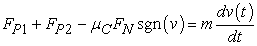(1)

where: FP1, FP2, μC, FN, v and m are propulsive force, propulsive force, Coulomb friction coefficient between segment and surface, normal force, velocity of segment, weight of segment, respectively. The propulsive force is dependent on the number of snake robot segments, what can be written as: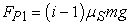(2)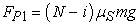(3)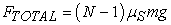(4)

where: N is the number of snake robot segments, i is number of moving segment, μS is static friction coefficient and g is gravitational acceleration. From these equations it is obvious that the higher number of snake robot segments is, the higher propulsive force the snake robot will has. The number of snake robot segments determines the number of phases of one locomotion cycle. By consideration that one locomotion cycle consists of N phases, the average velocity of snake robot is expressed by term: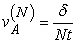(5)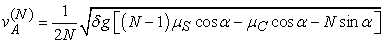(6)

where: α is angle of surface slope, t is duration of one phase of cycle and δ is a traveled distance of one snake robot segment. In the Figure 2 the average velocity of snake robot for different number of segments N is shown. The simulation were done for different angles of surface slope and for μS=0.5 and μC=0.3. The weight of one segment is 271 g.

In the Figure 2 one can see, that the snake robot has maximum average velocity with four segments. By further increasing of number of segments the velocity decreases.

Next analysis shows the propulsive force of snake robot with changing angle of surface slope and number of segments N.

It is clear that the higher number of segments is, the higher propulsive force the snake robot will has. Though, by increasing angle of surface slope the propulsive for decreases.

2.1. Optimal Number of Segments for Maximum Average Velocity

Of course, by increasing number of segments increases propulsive force affecting moving segment, but duration of one locomotion cycle decreases. Therefore it is necessary to express the optimal number of segments in order to obtain maximum average velocity. In other words, by suitable choice of number of segments one can obtain optimal average velocity of snake robot during its rectilinear locomotion. The optimal number of segments from the equation of average velocity is derived. By determination of local extreme from equation (6) we obtain: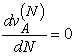(7)

The solution of equation (7) is term: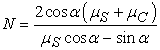(8)

From the equation (8) we can see, that optimal number of segments is dependent on the friction coefficients and angle of surface slope. By consideration of particular friction coefficients (by knowledge about surface and snake robot material) we can choose optimal number of segments. In the Figure 4 the equation (8) is shown with consideration of identical Coulomb and static friction coefficients.

The function for optimal number of segments has some difficulties. We can see in the Figure 4 that function increases for some point to extreme values, up to 500 segments. It is clear, that we have to avoid to these combinations of values of friction coefficients and angles of surface slope. It can be reached for example by suitable design solution.

### 3. Influence of Dry and Viscous Environment on Rectilinear Locomotion

The propulsive force of snake robot is generated due to static friction forces between static segments and surface, on which they are placed. The change of friction characteristic of surfaces results in different maximum propulsive force. From the equation (6), what is equation of motion for snake robot segment moving on dry surface, we can obtain term for velocity of moving segment: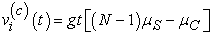(9)

In the case of viscous environment, the equation of velocity for moving segment is: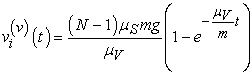(10)

In the Figure 5 Coulomb friction in comparison with viscous friction concerning snake robot segment velocity is shown. For simulation there were used following parameters: m=271 g, μC=0.15, μS=0.3, g=9.81 ms-2. During simulation was considered, that snake robot consists of 3 segments (N). The first segment is in the motion while other are at rest.

In the case of dry friction which by Coulomb friction model is represented, friction has the same value for arbitrary velocity. Therefore the segment velocity is not limited by friction. In the case of viscous surface the velocity of segment is limited by term: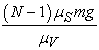(11)

The velocity of segment cannot overreach this value. The limitation of segment velocity for viscous surface can be seen in the Figure 5 by consideration of viscous friction coefficient μV = 0.5 Pa.s. Nevertheless, this value is rather theoretical, because viscous friction coefficient is usually lower order value.

### 4. Experimental Analysis of Snake Robot Segment Motion on Different Kinds of Surfaces

For experimental analysis there was used snake robot LocoSnake consisting of four segments. The experimental analysis is based on analysis of segment motion with consideration of different surfaces. During the experiment there are investigated motion properties of segment with three kind of surfaces, namely dry surface, viscous surface using an oil and viscous surface using lubricant. The measuring of friction in the Figure 6 is shown.

Dry surface is represented by clean pipe with square cross-section. The pipe is from polycarbonate. The viscous surface is represented by applying of oil and lubricant on surface of pipe. According to mathematical analysis concerning the optimal number of segments, the snake robot has the maximum average velocity using four segments (for the same Coulomb and static friction coefficients). Therefore for experiment is used LocoSnake consisting of only four segments.

The measurements by means of incremental linear sensor LARM MSL50 were done. The sensor serves for measuring of precise displacement up to 50 mm. LARM MSL50 has measuring step 5μm. The sensor is attached to head and rear of snake robot. During experiment only head segment moves while other segments are at rest in order to propulsive force can be generated. The first sensor measures displacement and velocity of head segment. From these measurements the friction characteristic will be done. The second sensor measures displacement of rear segment.

The results of dry friction measurement in the Figure 7 are shown.

The value of friction force for different velocities is almost constant what suggests on Coulomb friction model. During dry friction the friction magnitude never overreach certain value of friction and velocity of segment can freely increase.

In the Figure 8 the results from measuring of viscous friction are shown. In this case the viscous friction is represented by presence of oil on the surface of pipe.

From the Figure 8 it is obvious, that friction softly rise, what indicates viscous friction. The friction course does not start with zero position in y-axis but with certain value of friction. Therefore final friction model is combination of Coulomb and viscous friction model.

In the Figure 9 are the results from measurement where there was used the lubricant on the surface of pipe.

In the Figure 9 we can also see the combination of Coulomb and viscous friction model. It is a little bit different in comparison with the case of oil.

The propulsive force for head segment by equation (9) is expressed. Maximum propulsive force according equation (9) is in our case roughly 3.2 N. If this value is overreach, the other segments will move as well. LocoSnake has linear actuators which have limited supply voltage up to 10 V. Using this value of voltage the linear actuator gives propulsive force roughly 0.85 N. Therefore the maximum linear propulsive force of LocoSnake is limited by voltage supply not by number of static segments. Because of this limitation the rear sensor should measure zero displacement.

Now, in the Figure 10 the displacements of segment moving on particular surfaces are compared.

All the three courses of displacement are almost the same. By using thin layer of lubricant on the surface the segment reaches its required maximum protrusion a little bit later. While segment moves forward, before segment clusters a layer of lubricant what causes softly deceleration of segment, see Figure 11.

In the following figure, the course of propulsive force affecting moving segment is shown.

As can be seen, regardless of kind of surface, the propulsive force for all the three cases is almost the same. It means that propulsive force is significantly higher than friction force reacting against the motion of segment.

In the Figure 13 the course of electric power is shown.

Figure 13. Electric power consumption analysis also shows that for all three kinds of friction the system expends the same power.

### 5. Conclusion and Future Work

In the paper snake robot rectilinear locomotion was investigated. Snake robot locomotion is based on friction forces between robot and its environment. Snake robot can generated the propulsive force for moving segment due to friction forces between static segments of snake robot and the surface, on which robot moves. Therefore the study deals with particular friction models as well. In the paper the motion analysis was done with focus on determination of average velocity of snake robot consisting of N segments. By increasing number of segments increases also propulsive force for moving segment but average velocity decreases. Because of this fact, in the work the optimal number of segments for maximum average velocity is determined. It was found, that snake robot has maximum average velocity using four segments. It is true only in case when static friction coefficient equals to Coulomb friction coefficient with considering of dry friction. In case of dry friction, velocity of snake robot segment is not limited, because friction force never over reach certain value. Using snake robot in viscous environment it is different and velocity of segment is limited by value, which is by term (11) expressed.

The experimental analysis of three different surfaces were done, namely dry surface, surface with layer of oil and surface with layer of lubricant. The experimental study shows that change of surface did not have great influence on segment motion. Concerning the displacement of segment, the dry surface and surface with oil were almost the same. The surface with lubricant was a little bit different. It is because of clustering the layer of lubricant before segment during its motion. Concerning the electric power consumption, all the three surfaces have almost the same results again.

From the experimental results it is clear, that differences in motion between dry and viscous friction are not significant. In the case of lubricated surface the segment was a little bit decelerated. Experimental analysis also shows that snake robot rectilinear locomotion can be used for dry and viscous environments as well. It is questionable how thick layer can be on surface in order to achieve expected locomotion. This issue will be investigated in the future. Future work should also deals with feedback control of segments positions by means of microcontrollers. By this way snake robot will be partly autonomous using some distance sensors.

### Acknowledgement

The authors would like to thank to Slovak Grant Agency – project VEGA 1/1205/12 “Numerical modeling of mechatronic systems” and project VEGA 1/0937/12. This contribution is also result of the project APVV-0091-11 “Using of methods of experimental and numerical modeling for increasing of competitiveness and innovation of mechanical and mechatronical systems”.

### References

  Dowling, K. J. (1997), Limbless locomotion: Learning to crawl with a snake robot, Doctoral Thesis, Carnegie Mellon University, pp. 3-7.In article  Maity, A., Mandal, S. K., Mazumder, S., Gosh, S (2009), Serpentine robot: An overview of current status & prospect, 14th International conference on machines and mechanisms, pp. 275.In article  Jurišica, L., Duchoň, F. (2009), Triangulation of Mobile Robot Position with Detected Ingerent Environment Landmarks, International Journal of Mechanics and Control, Vol. 10, No. 2, pp. 15 - 25.In article  Transeth, A. A., Pettersen K. Y., Liljebäck, P. (2009), A survey on snake like robot modeling and locomotion, Robotica, Vol. 27, pp. 999 - 1015.In article CrossRef  Hirose, S. (1993), Biologically inspired robots, Snake-like locomotors and manipulators, Oxford: Oxford University press, pp. 2 - 13.In article  Hopkins, J. K., Spranklin, B. W., Gupta, S. K. (2009), A survey of snake-inspired robot designs. Bioninspiration and Biomimetics.In article  Gmiterko, A., Dovica, M., Kelemen, M., Fedák, V., Mlýnková, Z., (2002), In-pipe bristled micromachine, Advanced Motion Control, pp. 599 - 603.In article  Lissmann, H. W. (1949), Rectilinear locomotion in a snake, The Journal of Experimental Biology, pp. 368 - 379.In article  Ghanbari, A., Fakhrabadi, M. S., Rostami, A. (2009), Dynamics and GA-Based Optimization of rectilinear Snake Robot, Intelligent Robotics and Applications, pp. 613 - 622.In article  Chernousko, F. L. (2002), The optimum rectilinear motion of a two-masses system, Journal of Applied Mathematics and Mechanics, Vol. 66., pp. 1 - 7.In article CrossRef  Virgala, I. (2012), Mechatronic approach to design of system imitating locomotion of snake in limited spaces, Doctoral Thesis, Technical University of Košice.In article  Olsson, H., Ǻström, K. J., Canudas de Wit, C., Gäfvert, M, Lischinsky, P. (1998), Friction models and friction compensation, European journal of control, No. 4, pp. 176 - 195.In article